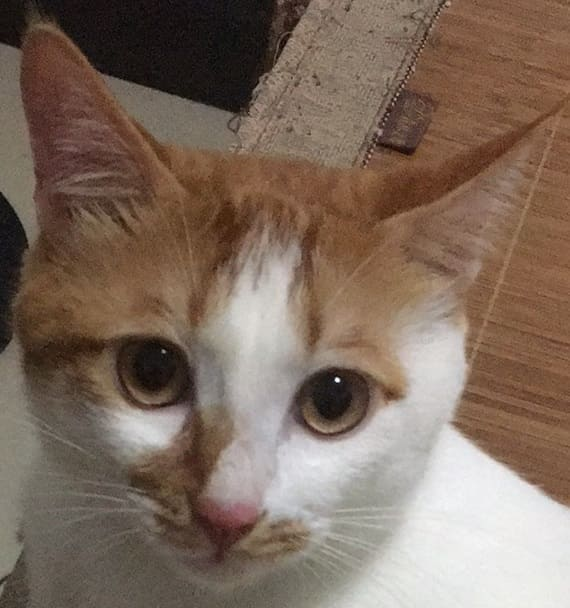OpenCV新建一个图片，并在图片上画由一点到另一点的直线，采用反走样形式

# 1 示例代码

#include <iostream>
#include <opencv2/core/core.hpp>
#include<opencv2/highgui/highgui.hpp>
using namespace cv;
using namespace std;
int main()
{
Mat M(400,200,CV_8UC1);//创建一个高400，宽200的灰度图的Mat对象
namedWindow("Test");     //创建一个名为Test窗口

for (int i=0;i<M.rows;i++)        //遍历每一行每一列并设置其像素值
{
for (int j=0;j<M.cols;j++)
{
M.at<uchar>(i,j)=255;
}
}
cv::Point p1;
p1.x=10;
p1.y=10;
cv::Point p2;
p2.x=100;
p2.y=200;
line(M,p1,p2,Scalar(0),2,CV_AA);//2为线的宽度，CV_AA为反走样形式
imshow("Test",M);   //窗口中显示图像
imwrite("E:/灰度图.jpg",M);    //保存生成的图片
waitKey(5000); //等待5000ms后窗口自动关闭
//  getchar();
}支付宝扫一扫微信扫一扫纵使晴明无雨色，入云深处亦沾衣。